## Minimization Of Boolean Expressions-

There are following two methods of minimizing or reducing the boolean expressions-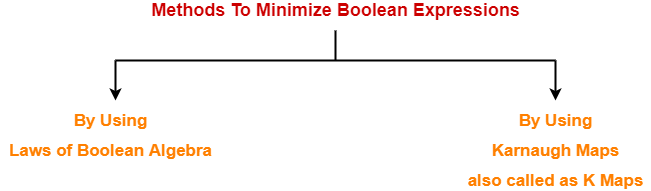1. By using laws of Boolean Algebra
2. By using Karnaugh Maps also called as K Maps

## Karnaugh Map-

 The Karnaugh Map also called as K Map is a graphical representation that provides a systematic method for simplifying the boolean expressions.

For a boolean expression consisting of n-variables, number of cells required in K Map = 2n cells.

### Two Variable K Map-

• Two variable K Map is drawn for a boolean expression consisting of two variables.
• The number of cells present in two variable K Map = 22 = 4 cells.
• So, for a boolean function consisting of two variables, we draw a 2 x 2 K Map.

Two variable K Map may be represented as-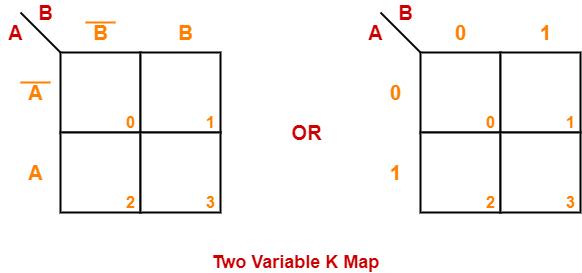Here, A and B are the two variables of the given boolean function.

### Three Variable K Map-

• Three variable K Map is drawn for a boolean expression consisting of three variables.
• The number of cells present in three variable K Map = 23 = 8 cells.
• So, for a boolean function consisting of three variables, we draw a 2 x 4 K Map.

Three variable K Map may be represented as-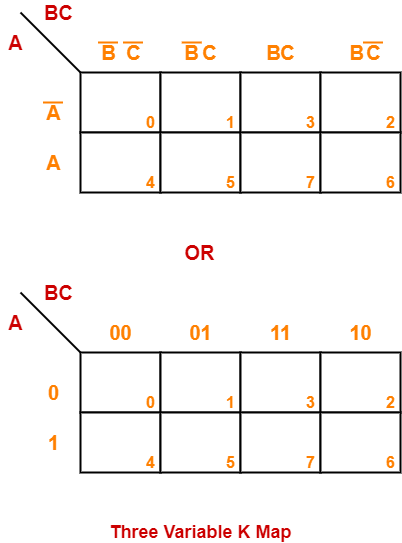Here, A, B and C are the three variables of the given boolean function.

### Four Variable K Map-

• Four variable K Map is drawn for a boolean expression consisting of four variables.
• The number of cells present in four variable K Map = 24 = 16 cells.
• So, for a boolean function consisting of four variables, we draw a 4 x 4 K Map.

Four variable K Map may be represented as-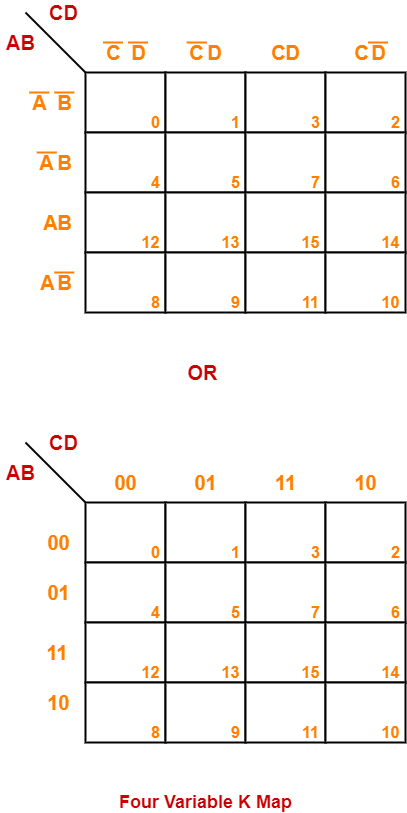Here, A, B, C and D are the four variables of the given boolean function.

## Karnaugh Map Simplification Rules-

To minimize the given boolean function,

• We draw a K Map according to the number of variables it contains.
• We fill the K Map with 0’s and 1’s according to its function.
• Then, we minimize the function in accordance with the following rules.

### Rule-01:

• We can either group 0’s with 0’s or 1’s with 1’s but we can not group 0’s and 1’s together.
• X representing don’t care can be grouped with 0’s as well as 1’s.

### NOTE

There is no need of separately grouping X’s i.e. they can be ignored if all 0’s and 1’s are already grouped.

### Rule-02:

• Groups may overlap each other.

### Rule-03:

• We can only create a group whose number of cells can be represented in the power of 2.
• In other words, a group can only contain 2n i.e. 1, 2, 4, 8, 16 and so on number of cells.

### Example-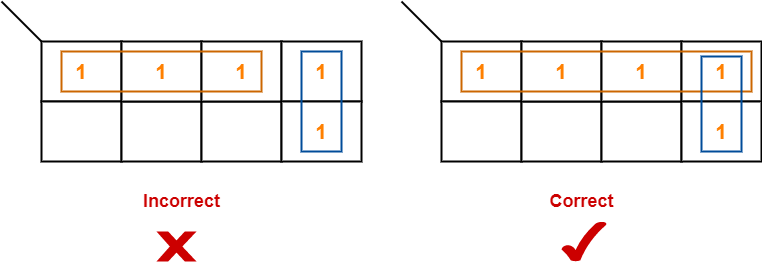### Rule-04:

• Groups can be only either horizontal or vertical.
• We can not create groups of diagonal or any other shape.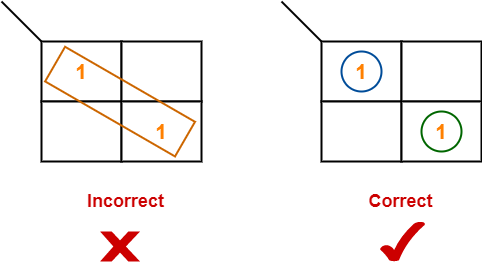### Rule-05:

• Each group should be as large as possible.

### Example-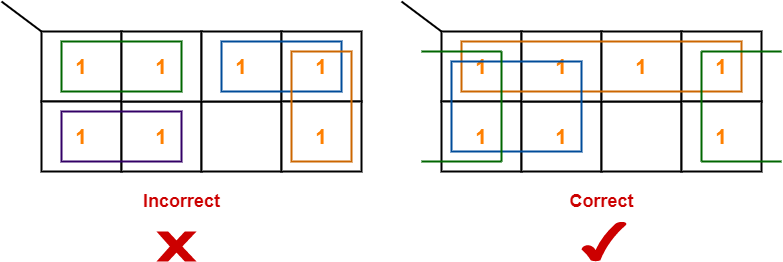### Rule-06:

• Opposite grouping and corner grouping are allowed.
• The example of opposite grouping is shown illustrated in Rule-05.
• The example of corner grouping is shown below.

### Example-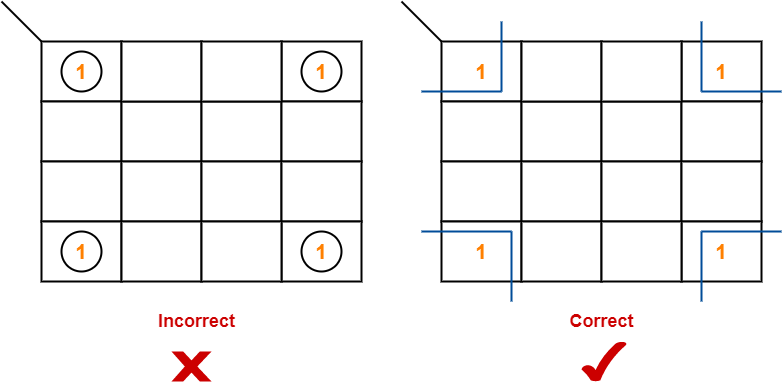### Rule-07:

• There should be as few groups as possible.

## Problem-01:

Minimize the following boolean function-

F(A, B, C, D) = Σm(0, 1, 2, 5, 7, 8, 9, 10, 13, 15)

## Solution-

• Since the given boolean expression has 4 variables, so we draw a 4 x 4 K Map.
• We fill the cells of K Map in accordance with the given boolean function.
• Then, we form the groups in accordance with the above rules.

Then, we have-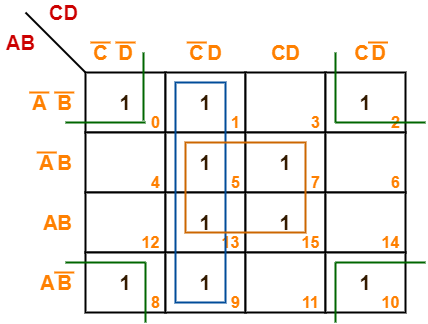Now,

F(A, B, C, D)

= (A’B + AB)(C’D + CD) + (A’B’ + A’B + AB + AB’)C’D + (A’B’ + AB’)(C’D’ + CD’)

= BD + C’D + B’D’

Thus, minimized boolean expression is-

F(A, B, C, D) = BD + C’D + B’D’

## Problem-02:

Minimize the following boolean function-

F(A, B, C, D) = Σm(0, 1, 3, 5, 7, 8, 9, 11, 13, 15)

## Solution-

• Since the given boolean expression has 4 variables, so we draw a 4 x 4 K Map.
• We fill the cells of K Map in accordance with the given boolean function.
• Then, we form the groups in accordance with the above rules.

Then, we have-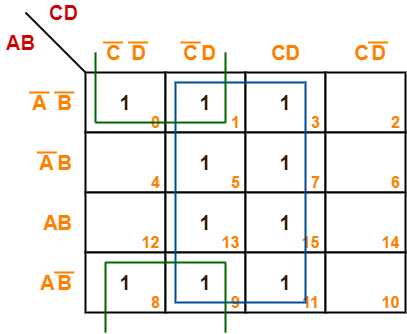Now,

F(A, B, C, D)

= (A’B’ + A’B + AB + AB’)(C’D + CD) + (A’B’ + AB’)(C’D’ + C’D)

= D + B’C’

Thus, minimized boolean expression is-

F(A, B, C, D) = B’C’ + D

## Problem-03:

Minimize the following boolean function-

F(A, B, C, D) = Σm(1, 3, 4, 6, 8, 9, 11, 13, 15) + Σd(0, 2, 14)

## Solution-

• Since the given boolean expression has 4 variables, so we draw a 4 x 4 K Map.
• We fill the cells of K Map in accordance with the given boolean function.
• Then, we form the groups in accordance with the above rules.

Then, we have-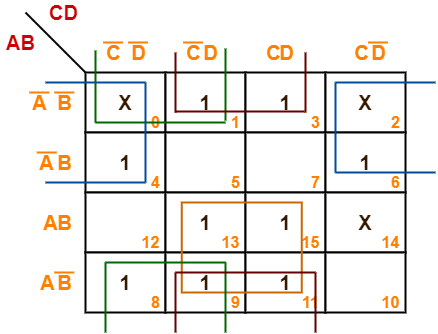Now,

F(A, B, C, D)

= (AB + AB’)(C’D + CD) + (A’B’ + AB’)(C’D + CD) + (A’B’ + AB’)(C’D’ + C’D) + (A’B’ + A’B)(C’D’ + CD’)

= AD + B’D + B’C’ + A’D’

Thus, minimized boolean expression is-

F(A, B, C, D) = AD + B’D + B’C’ + A’D’

## Problem-04:

Minimize the following boolean function-

F(A, B, C) = Σm(0, 1, 6, 7) + Σd(3, 5)

## Solution-

• Since the given boolean expression has 3 variables, so we draw a 2 x 4 K Map.
• We fill the cells of K Map in accordance with the given boolean function.
• Then, we form the groups in accordance with the above rules.

Then, we have-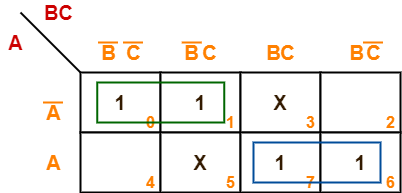Now,

F(A, B, C)

= A'(B’C’ + B’C) + A(BC + BC’)

= A’B’ + AB

Thus, minimized boolean expression is-

F(A, B, C) = AB + A’B’

### NOTE-

• It may be noted that there is no need of considering the quad group.
• This is because even if we consider that group, we will have to consider the other two duets.
• So, there is no use of considering that quad group.

## Problem-05:

Minimize the following boolean function-

F(A, B, C) = Σm(1, 2, 5, 7) + Σd(0, 4, 6)

## Solution-

• Since the given boolean expression has 3 variables, so we draw a 2 x 4 K Map.
• We fill the cells of K Map in accordance with the given boolean function.
• Then, we form the groups in accordance with the above rules.

Then, we have-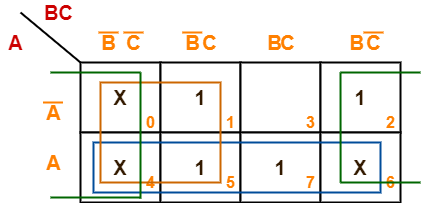Now,

F(A, B, C)

= (A + A’)(B’C’ + B’C) + A(B’C’ + B’C + BC + BC’) + (A + A’)(B’C’ + BC’)

= B’ + A + C’

Thus, minimized boolean expression is-

F(A, B, C) = A + B’ + C’

## Problem-06:

Minimize the following boolean function-

F(A, B, C) = Σm(0, 1, 6, 7) + Σd(3, 4, 5)

## Solution-

• Since the given boolean expression has 3 variables, so we draw a 2 x 4 K Map.
• We fill the cells of K Map in accordance with the given boolean function.
• Then, we form the groups in accordance with the above rules.

Then, we have-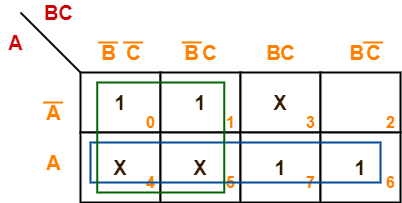Now,

F(A, B, C)

= (A + A’)(B’C’ + B’C) + A(B’C’ + B’C + BC + BC’)

= B’ + A

Thus, minimized boolean expression is-

F(A, B, C) = A + B’

## Problem-07:

Minimize the following boolean function-

F(A, B, C, D) = Σm(0, 2, 8, 10, 14) + Σd(5, 15)

## Solution-

• Since the given boolean expression has 4 variables, so we draw a 4 x 4 K Map.
• We fill the cells of K Map in accordance with the given boolean function.
• Then, we form the groups in accordance with the above rules.

Then, we have-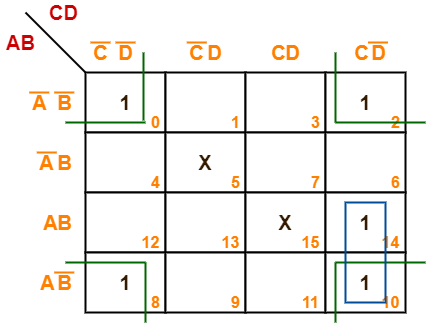Now,

F(A, B, C, D)

= (AB + AB’)CD’ + (A’B’ + AB’)(C’D’ + CD’)

= ACD’ + B’D’

Thus, minimized boolean expression is-

F(A, B, C, D) = ACD’ + B’D’

## Problem-08:

Minimize the following boolean function-

F(A, B, C, D) = Σm(3, 4, 5, 7, 9, 13, 14, 15)

## Solution-

• Since the given boolean expression has 4 variables, so we draw a 4 x 4 K Map.
• We fill the cells of K Map in accordance with the given boolean function.
• Then, we form the groups in accordance with the above rules.

Then, we have-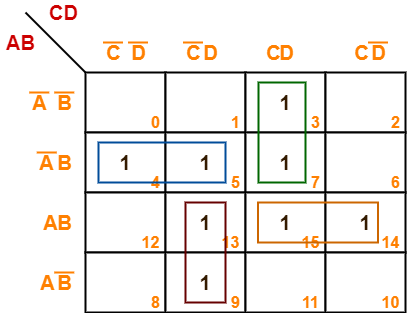Now,

F(A, B, C, D)

= A’B(C’D’ + C’D) + (A’B’ + A’B)(CD) + (AB + AB’)(C’D) + AB(CD + CD’)

= A’BC’ + A’CD + AC’D + ABC

Thus, minimized boolean expression is-

F(A, B, C, D) = A’BC’ + A’CD + AC’D + ABC

It is important to note that we are not considering the quad group because we have to consider the duets anyhow.

## Problem-09:

Consider the following boolean function-

F(W, X, Y, Z) = Σm(1, 3, 4, 6, 9, 11, 12, 14)

This function is independent ________ number of variables. Fill in the blank.

## Solution-

• Since the given boolean expression has 4 variables, so we draw a 4 x 4 K Map.
• We fill the cells of K Map in accordance with the given boolean function.
• Then, we form the groups in accordance with the above rules.

Then, we have-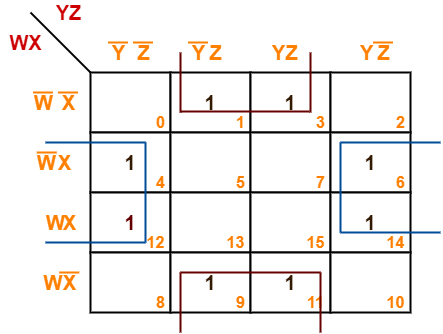Now,

F(W, X, Y, Z)

= (W’X + WX)(Y’Z’ + YZ’) + (W’X’ + WX’)(Y’Z + YZ)

= XZ’ + X’Z

= X ⊕ Z

Thus, minimized boolean expression is-

F(W, X, Y, Z) = X ⊕ Z

Clearly, the given boolean function depends on only two variables X and Z.

Hence, it is independent of other two variables W and Y.

Next Article- Neutral Functions

Get more notes and other study material of Digital Design.

Watch video lectures by visiting our YouTube channel LearnVidFun.